# Python | Plotting charts in excel sheet using openpyxl module | Set – 1

• Difficulty Level : Medium
• Last Updated : 04 Jul, 2022

Prerequisite: Reading & Writing to excel sheet using openpyxl Openpyxl is a Python library using which one can perform multiple operations on excel files like reading, writing, arithmetic operations and plotting graphs. Let’s see how to plot different charts using realtime data. Charts are composed of at least one series of one or more data points. Series themselves are comprised of references to cell ranges. For plotting the charts on an excel sheet, firstly, create chart object of specific chart class( i.e BarChart, LineChart etc.). After creating chart objects, insert data in it and lastly, add that chart object in the sheet object.

Code #1 : Plot the Bar Chart For plotting the bar chart on an excel sheet, use BarChart class from openpyxl.chart submodule.

## Python3

 `# import openpyxl module` `import` `openpyxl`   `# import BarChart class from openpyxl.chart sub_module` `from` `openpyxl.chart ``import` `BarChart,Reference`   `# Call a Workbook() function of openpyxl ` `# to create a new blank Workbook object` `wb ``=` `openpyxl.Workbook()`   `# Get workbook active sheet ` `# from the active attribute.` `sheet ``=` `wb.active`   `# write o to 9 in 1st column of the active sheet` `for` `i ``in` `range``(``10``):` `    ``sheet.append([i])`   `# create data for plotting` `values ``=` `Reference(sheet, min_col ``=` `1``, min_row ``=` `1``,` `                         ``max_col ``=` `1``, max_row ``=` `10``)`   `# Create object of BarChart class` `chart ``=` `BarChart()`   `# adding data to the Bar chart object` `chart.add_data(values)`   `# set the title of the chart` `chart.title ``=` `" BAR``-``CHART "`   `# set the title of the x-axis` `chart.x_axis.title ``=` `" X_AXIS "`   `# set the title of the y-axis` `chart.y_axis.title ``=` `" Y_AXIS "`   `# add chart to the sheet` `# the top-left corner of a chart` `# is anchored to cell E2 .` `sheet.add_chart(chart, "E2")`   `# save the file` `wb.save("barChart.xlsx")`

Output: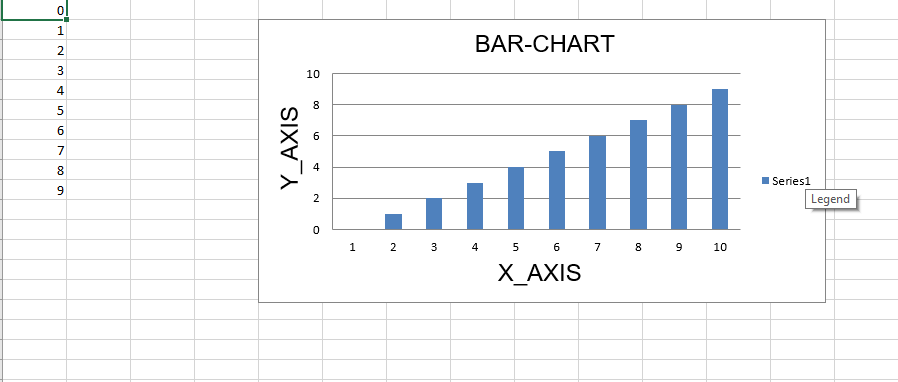Code #2 : Plot the 3D Bar Chart For plotting the 3D bar chart on an excel sheet, use BarChart3D class from openpyxl.chart submodule.

## Python3

 `# import openpyxl module` `import` `openpyxl`   `# import BarChart3D class from openpyxl.chart sub_module` `from` `openpyxl.chart ``import` `BarChart3D,Reference`   `# write o to 9 in 1st column of the active sheet` `for` `i ``in` `range``(``10``):` `    ``sheet.append([i])`   `values ``=` `Reference(sheet, min_col ``=` `1``, min_row ``=` `1``,` `                         ``max_col ``=` `1``, max_row ``=` `10``)`   `# Create object of BarChart3D class` `chart ``=` `BarChart3D()`   `chart.add_data(values)`   `# set the title of the chart` `chart.title ``=` `" BAR``-``CHART3D "`   `# set the title of the x-axis` `chart.x_axis.title ``=` `" X AXIS "`   `# set the title of the y-axis` `chart.y_axis.title ``=` `" Y AXIS "`   `# add chart to the sheet` `# the top-left corner of a chart` `# is anchored to cell E2.` `sheet.add_chart(chart, "E2")`   `# save the file` `wb.save("BarChart3D.xlsx")`

Output: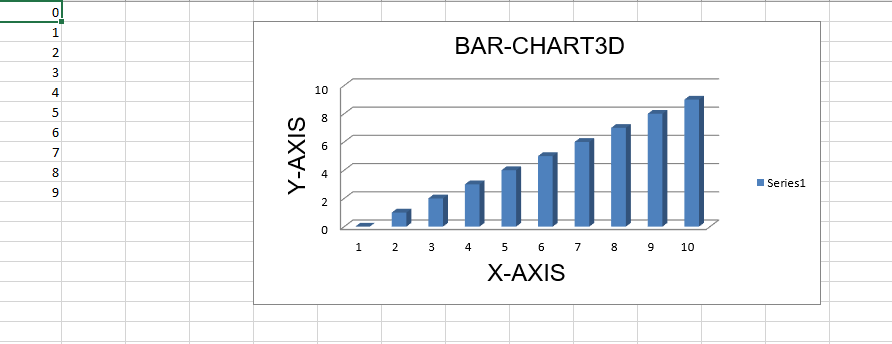Code #3 : Plot the Area Chart For plotting the Area chart on an excel sheet, use AreaChart class from openpyxl.chart submodule.

## Python3

 `import` `openpyxl`   `# import AreaChart class from openpyxl.chart sub_module` `from` `openpyxl.chart ``import` `AreaChart,Reference`     `wb ``=` `openpyxl.Workbook()` `sheet ``=` `wb.active`   `# write o to 9 in 1st column of the active sheet` `for` `i ``in` `range``(``10``):` `    ``sheet.append([i])`   `values ``=` `Reference(sheet, min_col ``=` `1``, min_row ``=` `1``,` `                         ``max_col ``=` `1``, max_row ``=` `10``)`   `# create object of AreaChart class` `chart ``=` `AreaChart()`   `chart.add_data(values)`   `# set the title of the chart` `chart.title ``=` `" AREA``-``CHART "`   `# set the title of the x-axis` `chart.x_axis.title ``=` `" X``-``AXIS "`   `# set the title of the y-axis` `chart.y_axis.title ``=` `" Y``-``AXIS "`   `# add chart to the sheet` `# the top-left corner of a chart` `# is anchored to cell E2 .` `sheet.add_chart(chart, "E2")`   `# save the file` `wb.save("AreaChart.xlsx")`

Output: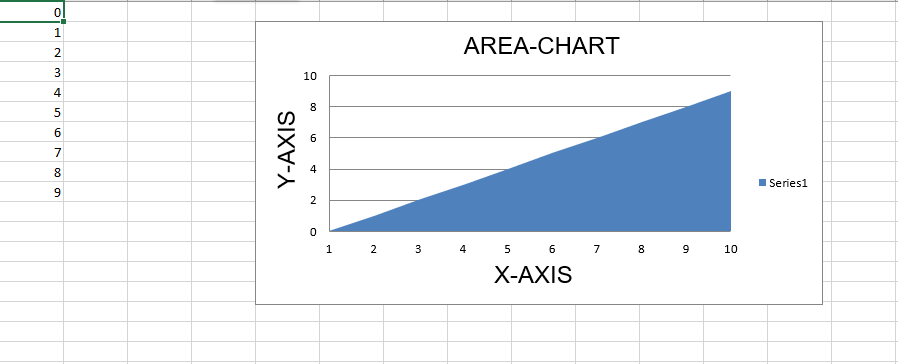Code #4 : Plot the 3D Area Chart For plotting the 3D Area chart on an excel sheet, use AreaChart3D class from openpyxl.chart submodule.

## Python3

 `import` `openpyxl`   `# import AreaChart3D class from openpyxl.chart sub_module` `from` `openpyxl.chart ``import` `AreaChart3D,Reference`   `wb ``=` `openpyxl.Workbook()` `sheet ``=` `wb.active`   `# write o to 9 in 1st column of the active sheet` `for` `i ``in` `range``(``10``):` `    ``sheet.append([i])`   `values ``=` `Reference(sheet, min_col ``=` `1``, min_row ``=` `1``,` `                         ``max_col ``=` `1``, max_row ``=` `10``)`   `# Create object of AreaChart3D class` `chart ``=` `AreaChart3D()`   `chart.add_data(values)`   `# set the title of the chart` `chart.title ``=` `" AREA``-``CHART3D "`   `# set the title of the x-axis` `chart.x_axis.title ``=` `" X``-``AXIS "`   `# set the title of the y-axis` `chart.y_axis.title ``=` `" Y``-``AXIS "`   `# add chart to the sheet` `# the top-left corner of a chart` `# is anchored to cell E2 .` `sheet.add_chart(chart, "E2")`   `# save the file` `wb.save("AreaChart3D.xlsx")`

Output: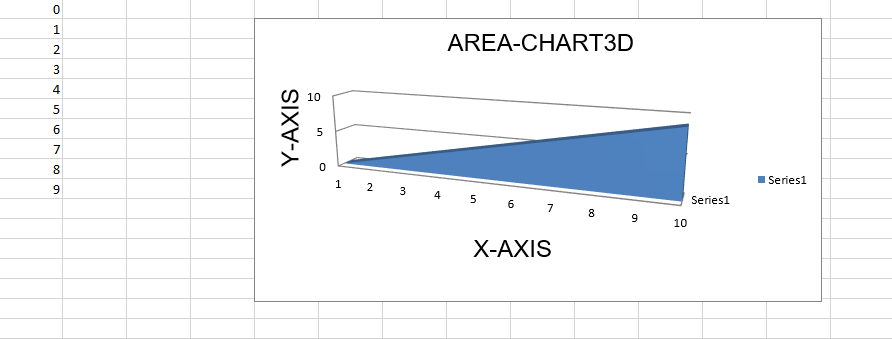Code #5 : Plot a Line Chart. For plotting the Line chart on an excel sheet, use LineChart class from openpyxl.chart submodule.

## Python3

 `# import openpyxl module` `import` `openpyxl`   `# import LineChart class from openpyxl.chart sub_module` `from` `openpyxl.chart ``import` `LineChart,Reference`   `wb ``=` `openpyxl.Workbook()` `sheet ``=` `wb.active`   `# write o to 9 in 1st column of the active sheet` `for` `i ``in` `range``(``10``):` `    ``sheet.append([i])`   `values ``=` `Reference(sheet, min_col ``=` `1``, min_row ``=` `1``,` `                         ``max_col ``=` `1``, max_row ``=` `10``)`   `# Create object of LineChart class` `chart ``=` `LineChart()`   `chart.add_data(values)`   `# set the title of the chart` `chart.title ``=` `" LINE``-``CHART "`   `# set the title of the x-axis` `chart.x_axis.title ``=` `" X``-``AXIS "`   `# set the title of the y-axis` `chart.y_axis.title ``=` `" Y``-``AXIS "`   `# add chart to the sheet` `# the top-left corner of a chart` `# is anchored to cell E2 .` `sheet.add_chart(chart, "E2")`   `# save the file` `wb.save("LineChart.xlsx")`

Output: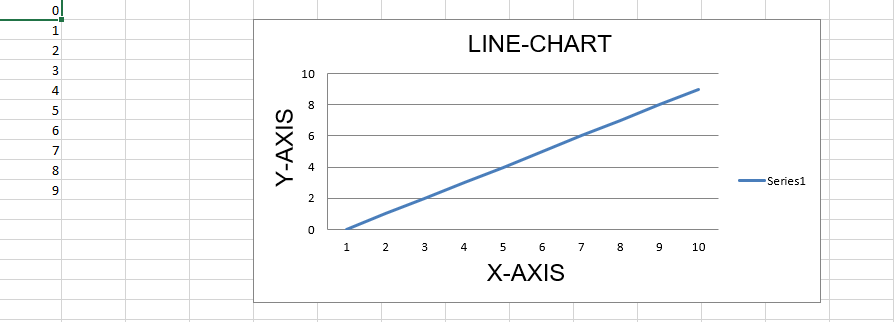Code #6 : Plot a 3D Line Chart. For plotting the 3D Line chart on an excel sheet we have to use LineChart3D class from openpyxl.chart submodule.

## Python3

 `import` `openpyxl`   `# import LineChart3D class from openpyxl.chart sub_module` `from` `openpyxl.chart ``import` `LineChart3D,Reference`   `wb ``=` `openpyxl.Workbook()` `sheet ``=` `wb.active`   `# write o to 9 in 1st column of the active sheet` `for` `i ``in` `range``(``10``):` `    ``sheet.append([i])`   `values ``=` `Reference(sheet, min_col ``=` `1``, min_row ``=` `1``,` `                         ``max_col ``=` `1``, max_row ``=` `10``)`   `# Create object of LineChart3D class` `chart ``=` `LineChart3D()`   `chart.add_data(values)`   `# set the title of the chart` `chart.title ``=` `" LINE``-``CHART3D "`     `# set the title of the x-axis` `chart.x_axis.title ``=` `" X``-``AXIS "`   `# set the title of the y-axis` `chart.y_axis.title ``=` `" Y``-``AXIS "`   `# add chart to the sheet` `# the top-left corner of a chart` `# is anchored to cell E2 .` `sheet.add_chart(chart, "E2")`   `# save the file` `wb.save("LineChart3D.xlsx")`

Output: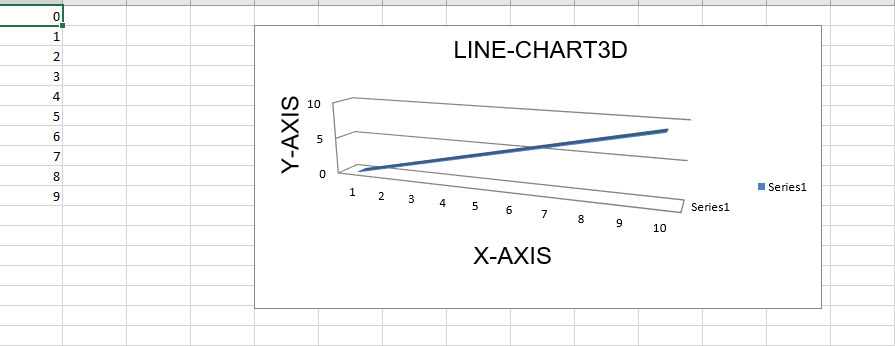My Personal Notes arrow_drop_up
Recommended Articles
Page :